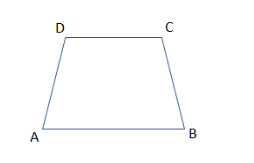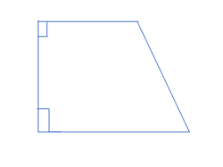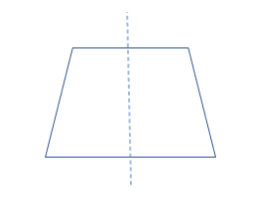QuestionAnswers

# A trapezium in which no parallel sides are equal is said to be(a) Right Trapezium(b) Equilateral Trapezium(c) Isosceles Trapezium(d) None of these

Hint: First, before proceeding for this, we must know the various types of trapezium and also the basic definition of the trapezium. Then, trapezium is defined as quadrilateral with a pair of parallel sides or a parallelogram is called a trapezium if it has two parallel sides. Then, after checking all the conditions for the different types of trapezium, we get the correct answer.

In this question, we are supposed to find a trapezium in which no parallel sides are equal is called what kind of trapezium.
So, before proceeding for this, we must know the various types of trapezium and also the basic definition of the trapezium.
So, trapezium is defined as quadrilateral with a pair of parallel sides.
Also, a parallelogram is called a trapezium if it has two parallel sides.
So, a trapezium ABCD is formed with parallel sides as AB and CD.Now, the first type of trapezium is right trapezium which has at least two right angles as shown in the figure:Then, the second type of trapezium is equilateral trapezium which is not possible as if it has all equal sides then it becomes a rhombus and contradicts the condition of the trapezium.
Then, the third type of trapezium is isosceles trapezium in which two sides are opposite and parallel but no parallel sides are equal. It has the property that there is a line bisecting one pair of opposite sides as:So, from the above condition, a trapezium in which no parallel sides are equal is said to be isosceles trapezium.
So, the correct answer is “Option C”.

Note: Now, to solve these types of questions we need to be careful of the facts of the shapes in the geometry to get the correct answer to these types of questions. As sometimes some options are given for confusion only like the option as equilateral trapezium as it can’t exist in reality due to the property of trapezoid. So, be careful about the facts mentioned above.